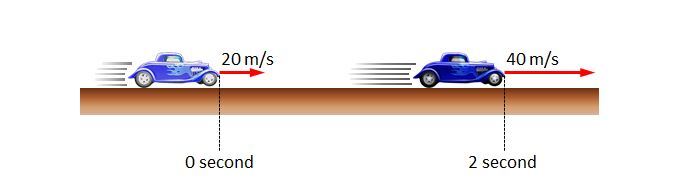# Accelerating carThe above picture depicts a car, which was moving on a straight line with a velocity of $20$ m/s, is now speeding up for $2$ seconds, raising the velocity up to $40$ m/s. If the rate of change of the velocity of the car is constant, then what are the acceleration and the moving distance during the $2$ seconds, respectively?

×